Algebra II : Multiplying and Dividing Radicals

Example Questions

← Previous 1 3 4 5 6 7

Example Question #331 : College Algebra

Multiply and express the answer in the simplest form: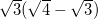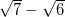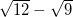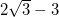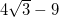Explanation: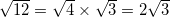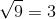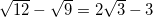Example Question #1 : Multiplying And Dividing Radicals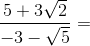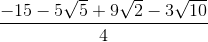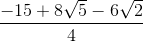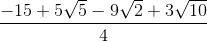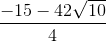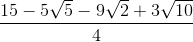Explanation: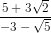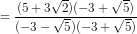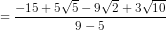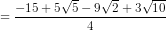Example Question #2 : Multiplying And Dividing Radicals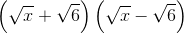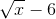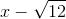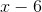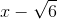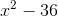Explanation:

FOIL with difference of squares.  The multiplying cancels the square roots on both terms.

Example Question #52 : Review And Other Topics

Simplify.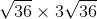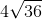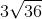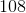Explanation:

We can solve this by simplifying the radicals first: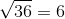Plugging this into the equation gives us: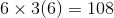Example Question #3 : Multiplying And Dividing Radicals

Simplify.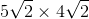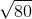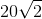Explanation:

Note: the product of the radicals is the same as the radical of the product: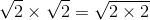which is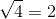Once we understand this, we can plug it into the equation: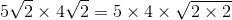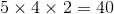Example Question #4 : Multiplying And Dividing Radicals

Simplify.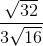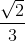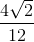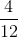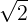Explanation:

We can simplify the radicals: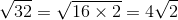and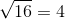Plug in the simplifed radicals into the equation: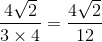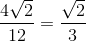Example Question #5 : Multiplying And Dividing Radicals

Simplify and rationalize the denominator if needed,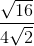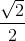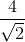Explanation:

We can only simplify the radical in the numerator:Plugging in the simplifed radical into the equation we get: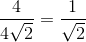Note: We simplified further because both the numerator and denominator had a "4" which canceled out.

Now we want to rationalize the denominator,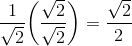Example Question #6 : Multiplying And Dividing Radicals

Simplify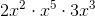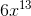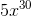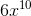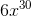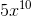Explanation:

To simplify, you must use the Law of Exponents.

First you must multiply the coefficients then add the exponents: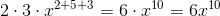Example Question #53 : Review And Other Topics

What is the product of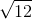and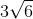?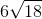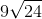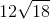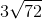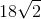Explanation:

First, simplify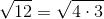to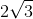.

Then set up the multiplication problem: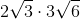.

Multiply the terms outside of the radical, then the terms under the radical: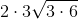then simplfy: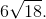The radical is still not in its simplest form and must be reduced further: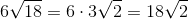. This is the radical in its simplest form.

Example Question #7 : Multiplying And Dividing Radicals

Simplify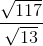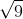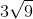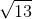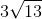Explanation:

To divide the radicals, simply divide the numbers under the radical and leave them under the radical: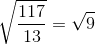Then simplify this radical:.

← Previous 1 3 4 5 6 7

All Algebra II Resources